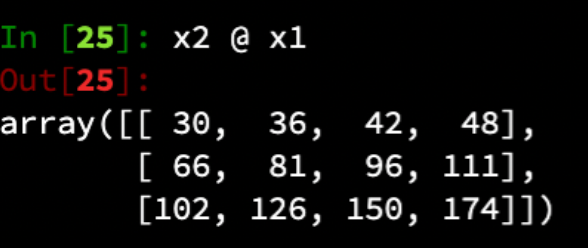# Matrix Multiplication

Even I Cant’ Come Up With a Way to Try to Make This Entertaining

Sometimes, you just gotta eat your vegetables.

In my next post, we’re going to go into some math behind forward propogation in neural networks. But before we do that, I need to make sure we’re all on the same page when it comes to one of the most important math concepts in all of machine learning: matrix multiplication, and in particular, calculating inner products.

Let’s start by defining two matrices, x1 and x2, as follows:

I will use the numpy @ designation to signify that an inner product calculation is being performed (or at least requested); for example x1 @ x2.

If we want to calculate the inner product of these two matrices, we’re going to need to understand some rules — the first one being about the shapes. Our matrix x1 has a shape of (3, 4), meaning 3 rows (or observations) and 4 columns (or features / variables). Our x2 matrix has a shape of (3, 3). In order to calculate an inner product between these two, we have to make sure the value of the second shape value in our first matrix matches the value of the first shape value in the second one. In other words, the inner shape values have to match for this to work.

What that means is this: if I tried to run x1 @ x2, I’m trying to define an inner product between x1 (shape 3, 4) and x2 (shape 3,3). Rewriting just a bit, we’re requesting (3, 4) @ (3, 3). Take a look at those inner values — the second shape value in the first matrix is a 4, and the first value in the second matrix is a 3. Therefore, trying to run x1 @ x2 is going to return an error.

There are a couple of ways we could mitigate this. The first would be to use numpy’s transpose function, which can be called by simply adding “.T” to the end of a matrix. Transposing basically inverts a matrix so that it’s rows become columns and columns become rows. If we tried x1.T @ x2, this would formulate shape-wise as (4, 3) @ (3, 3). And now, since the inner numbers match, we would get a result. Alternatively, we could reorder x1 and x2 and simply put x2 first. If we tried x2 @ x1, that formulates shape-wise as (3, 3) @ (3, 4). Once again, the inner values match, so an inner product can be calculated.Sometimes the hardest part of success is trying to figure out what it all means…

So where did these values come from? Basically, an inner product is the multiplication of the values in every row in the first matrix by their accompanying values in the column of the second matrix. Let’s take a look at how this actually works.

We start by taking the first value in the first row times the first value in the first column, then add that to the product of the second value of each, and then again the third. The math for the first result in our answer matrix goes like this:

This gives us the first value in the first row of our resulting matrix. For the next result, we once again use the first row in the first matrix, but this time multiply the values by the corresponding values in the second column, which gives us the second value in our resulting matrix. We repeat once again using the first row of the first matrix, but now using the third column of the second matrix, and then finally one more time subsituting the fourth column of the second matrix. This produces four values that correspond to the first row in the first matrix, and so are output as follows:

This process repeats for the second row of the first matrix:

And finally for the third row:

# Why This Matters

It matters that you understand this because this kind of matrix multiplication shows up in a *lot* of machine learning. While I’ve tried to avoid using the matrix notation when discussing math concepts, when we dive into math for deep learning, using matrix multiplication techniques will allow us to vastly simplify the process for performing forward propogation — both from a pure number of calculations to perform, and in particular, the step in the process required to account for the bias / offset values along the way. I’ll explain it more in the next post, but in order to really get what’s going on, you need to understand what’s happening when you calculate an inner product between two matrices.

Now go get some dessert. You’ve earned it.

--

--